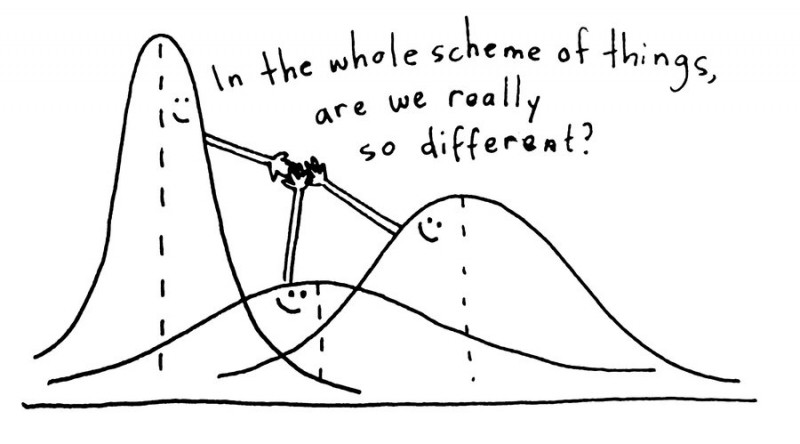# ANOVA

### ‘Analysis Of Variance – ANOVAAnalysis of variance (ANOVA) is an analysis tool used in statistics that splits the aggregate variability found inside a data set into two parts: systematic factors and random factors. The systematic factors have a statistical influence on the given data set, but the random factors do not. Analysts use the analysis of the variance test to determine the result that independent variables have on the dependent variable amid a regression study.

The analysis of variance test is the initial step in analyzing factors that affect a given data set. Once the analysis of variance test is finished, an analyst performs additional testing on the methodical factors that measurably contribute to the data set’s inconsistency. The analyst utilizes the analysis of the variance test results in an f-test to generate additional data that aligns with the proposed regression models.

The test allows comparison of more than two groups at the same time to determine whether a relationship exists between them. The test analyzes multiple groups to determine the types between and within samples. For example, a researcher might test students from multiple colleges to see if students from one of the colleges consistently outperform the others. Also, an R&D researcher might test two different processes of creating a product to see if one process is better than the other in terms of cost efficiency.

### Types of ANOVA

There are two types of analysis of variance: one-way (or unidirectional) and two-way. One-way or two-way refers to the number of independent variables in your Analysis of Variance test.

A one-way ANOVA evaluates the impact of a sole factor on a sole response variable. It determines whether all the samples are the same. The one-way ANOVA is used to determine whether there are any statistically significant differences between the means of three or more independent (unrelated) groups.

A two-way ANOVA is an extension of the one-way ANOVA. With a one-way, you have one independent variable affecting a dependent variable. With a two-way ANOVA, there are two independents. For example, a two-way ANOVA allows a company to compare worker productivity based on two independent variables, say salary and skill set. It is utilized to observe the interaction between the two factors. It tests the effect of two factors at the same time.

## 2 thoughts on “ANOVA”

error: Content is protected !!